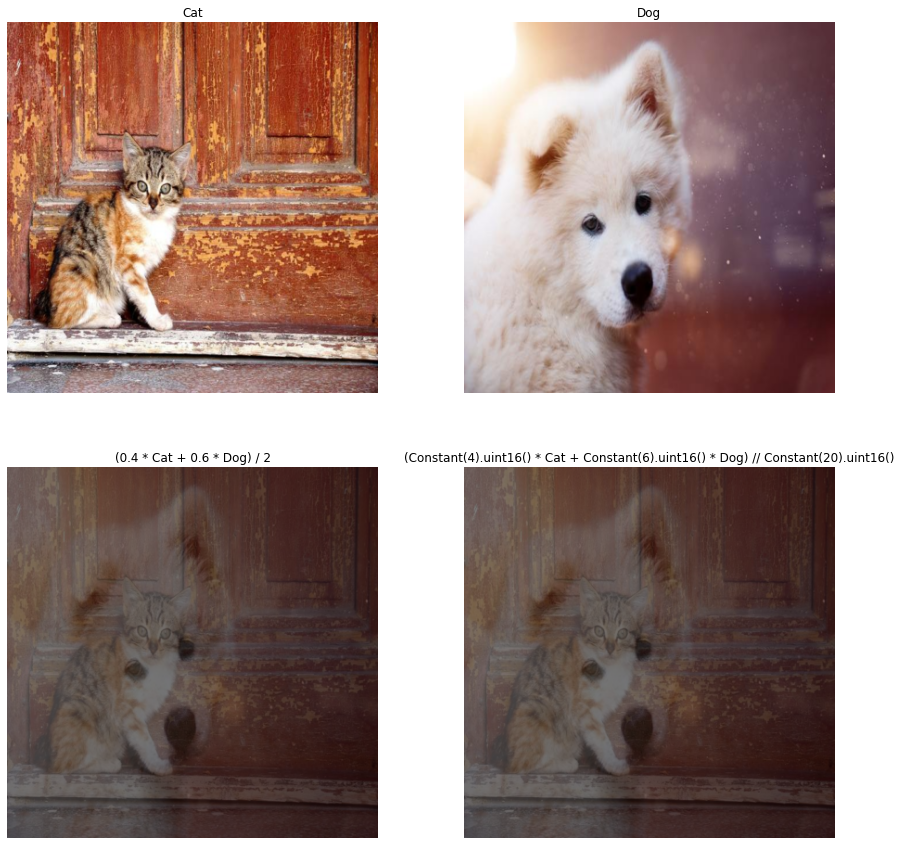# Custom Augmentations with Arithmetic Operations¶

This section shows you how to implement a custom augmentation by using expressions with arithmetic operations in the DALI Pipeline.

## Blending Images¶

We will create a pipeline that blends images in a few different ways. To easily visualize the results, we created the following file lists that contain pictures of cats and dogs:

• cats.txt

• dogs.txt

### Imports¶

:

from nvidia.dali.pipeline import Pipeline
import nvidia.dali.fn as fn
import nvidia.dali.types as types
from nvidia.dali.types import Constant


#### Explicitly Used Operators¶

1. The pipeline will use two readers.file to create two batches of tensors, one with cats and one with dogs.

2. We also need an decoders.image to decode the loaded images.

3. You need the resize operator for both inputs.

4. The arithmetic operators apply pointwise operations between tensors and require them to have matching shapes and sizes.

For this example, 400 x 400 images have been used.

5. The final operator that we may want to declare in the pipeline is a Cast operator to convert the data back into desired type.

#### The Graph with Custom Augmentation¶

Here are initial steps:

2. Decode both inputs.

3. Resize the inputs to equal sizes.

Now, we have two variables dogs and cats that represent two batches of equal-sized images. We can blend those images with some weights and reduce the pixel intensities by half by using this formula:

(0.4 * cats + 0.6 * dogs) / 2


Here, we used Python immediate values as the constant inputs in the arithmetic expression.

### Using dali.types.Constant to Indicate the Type¶

1. We can also be more careful about the types that we use, and do all of the computations in uint16.

The inputs are in uint8, and doing the computations with a constant that is marked as uint16 promotes the results to uint16. See the “DALI binary arithmetic operators - type promotions” tutorial for more information.

1. We can also use the // division that allows us to keep the integer type of the result.

(Constant(4).uint16() * cats + Constant(6).uint16() * dogs) // Constant(20).uint16()

1. We return both of the inputs and the results have been casted back to uint8.

:

pipe = Pipeline(batch_size=1, num_threads=4, device_id=0, seed=42)
with pipe:
cats_jpegs, _ = fn.readers.file(device="cpu", file_root="../../data/images", file_list="cats.txt")
dogs_jpegs, _ = fn.readers.file(device="cpu", file_root="../../data/images", file_list="dogs.txt")
images = fn.decoders.image([cats_jpegs, dogs_jpegs], device="cpu", output_type=types.RGB)
cats, dogs = fn.resize(images, resize_x=400, resize_y=400)
blend_float = (0.4 * cats + 0.6 * dogs) / 2
blend_uint16 = (Constant(4).uint16() * cats + Constant(6).uint16() * dogs) // Constant(20).uint16()

pipe.set_outputs(
cats,
dogs,
fn.cast_like(blend_float, cats),
fn.cast_like(blend_uint16, cats))


#### Running the Pipeline¶

1. Create an instance of the pipeline and build it. We use batch_size = 1 for simplicity of showing the result.

:

pipe.build()

1. We will use a simple helper function to show the images.

For larger batches, data_idx can be adjusted to show different samples. The output_titles will be used to set the titles of the pipeline output.

:

import matplotlib.pyplot as plt

def display(output, titles, cpu = True):
data_idx = 0
fig, axes = plt.subplots(len(output) // 2, 2, figsize=(15, 15))
if len(output) == 1:
axes = [axes]
for i, out in enumerate(output):
img = out.at(data_idx) if cpu else out.as_cpu().at(data_idx)
axes[i // 2, i % 2].imshow(img);
axes[i // 2, i % 2].axis('off')
axes[i // 2, i % 2].set_title(titles[i])

output_titles = [
"Cat", "Dog",
"(0.4 * Cat + 0.6 * Dog) / 2",
"(Constant(4).uint16() * Cat + Constant(6).uint16() * Dog) // Constant(20).uint16()"]

1. We will run and display the results.

You can play this cell several times to see the result for different images.

:

output = pipe.run()
display(output, output_titles)